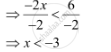# Solve the Given Inequality for Real X: 3x – 7 > 5x – 1 - Mathematics

Solve the given inequality for real x: 3x – 7 > 5x – 1

#### Solution

3x – 7 > 5x – 1

⇒ 3x – 7 + 7 > 5x – 1 + 7

⇒ 3x > 5x + 6

⇒ 3x – 5x > 5x + 6 – 5x

⇒ – 2> 6Thus, all real numbers x,which are less than –3, are the solutions of the given inequality.

Hence, the solution set of the given inequality is (–∞, –3).

Concept: Algebraic Solutions of Linear Inequalities in One Variable and Their Graphical Representation
Is there an error in this question or solution?

#### APPEARS IN

NCERT Class 11 Mathematics
Chapter 6 Linear Inequalities
Q 6 | Page 122